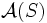# Thompson's replacement theorem for elementary abelian subgroups

View a complete list of replacement theorems| View a complete list of failures of replacement
This article states and (possibly) proves a fact that is true for odd-order p-groups: groups of prime power order where the underlying prime is odd. The statement is false, in general, for groups whose order is a power of two.
View other such facts for p-groups|View other such facts for finite groups

## Statement

### For an odd prime

Suppose$S$ is a group of prime power order for an odd prime$p$.

Let$\mathcal{A}(S)$ denote the set of all elementary abelian subgroups of maximum order in$S$ (i.e.,$|A| \ge |B|$ for all elementary abelian subgroups$B$ of$S$).

Suppose$A \in \mathcal{A}_e(S)$ and$B$ is an abelian subgroup such that$A$ normalizes$B$ but$B$ does not normalize$A$. Then, there exists an elementary abelian subgroup$A^*$ of$AB$ such that:

•$|A^*| = |A|$, so in particular,$A^* \in \mathcal{A}_e(S)$.
•$A \cap B$ is a proper subgroup of$A^* \cap B$.
•$A^*$ normalizes$A$.

### For the prime$2$

A slight modification works for the prime$2$, but we have to drop the requirement that$A^*$ be elementary abelian and instead only have$A^*$ abelian but of size at least that of$A$:

Let$\mathcal{A}(S)$ denote the set of all elementary abelian subgroups of maximum order in$S$ (i.e.,$|A| \ge |B|$ for all elementary abelian subgroups$B$ of$S$).

Suppose$A \in \mathcal{A}_e(S)$ and$B$ is an abelian subgroup such that$A$ normalizes$B$ but$B$ does not normalize$A$. Then, there exists an abelian subgroup$A^*$ of$AB$ such that:

•$|A^*| \ge |A|$.
•$A \cap B$ is a proper subgroup of$A^* \cap B$.
•$A^*$ normalizes$A$.